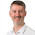## Friday, 28 November 2014

### Mini chart for alerts, text objects and popups

I was handed and interesting challenge yesterday.  The challenger was looking for a way to present both a list of dimensions, and an associated calculation, in a popup dialog.  This sounds like it should be quite achievable, but I couldn't think of an answer immediately!

We can, of course, present the list of dimensions using Concat:

=Concat(Customer, chr(10))

=Concat(Customer, chr(10), -Aggr(Sum(LineValue),Customer))

If we tried to add the Sum into the Concat's first parameter, we would get an error about not allowing an aggregation.

We were racking our brains to come up with a way to add the value (without seeing the bleeding obvious! - see below).  Eventually, I came up with this horrendous expression:

=If(Len(FirstSortedValue(Customer, -Aggr(Sum(LineValue), Customer),1))>0,
FirstSortedValue(Customer, -Aggr(Sum(LineValue), Customer),1)
& ' - ' & Num(Sum({} LineValue), '#,##0')
& chr(10), Null())

& If(Len(FirstSortedValue(Customer, -Aggr(Sum(LineValue), Customer),2))>0,
FirstSortedValue(Customer, -Aggr(Sum(LineValue), Customer),2)
& ' - ' & Num(Sum({} LineValue), '#,##0')
& chr(10), Null())

& If(Len(FirstSortedValue(Customer, -Aggr(Sum(LineValue), Customer),3))>0,
FirstSortedValue(Customer, -Aggr(Sum(LineValue), Customer),3)
& ' - ' & Num(Sum({} LineValue), '#,##0')
& chr(10), Null())

& If(Len(FirstSortedValue(Customer, -Aggr(Sum(LineValue), Customer),4))>0,
FirstSortedValue(Customer, -Aggr(Sum(LineValue), Customer),4)
& ' - ' & Num(Sum({} LineValue), '#,##0')
& chr(10), Null())

& If(Len(FirstSortedValue(Customer, -Aggr(Sum(LineValue), Customer),5))>0,
FirstSortedValue(Customer, -Aggr(Sum(LineValue), Customer),5)
& ' - ' & Num(Sum({} LineValue), '#,##0')
, Null())

This just shows the top 5 - but only if there is no match on the expression - in which case it would hide the matching values (because firstsortedvalue returns null).  So I had some thoughts and came up with this:

=If(Len(FirstSortedValue(DISTINCT Customer, -Aggr(Sum(LineValue)+Rand(), Customer),1))>0,
FirstSortedValue(DISTINCT Customer, -Aggr(Sum(LineValue)+Rand(), Customer),1)
& ' - ' & Num(Sum({} LineValue), '#,##0')
& chr(10), Null())

& If(Len(FirstSortedValue(DISTINCT Customer, -Aggr(Sum(LineValue)+Rand(), Customer),2))>0,
FirstSortedValue(DISTINCT Customer, -Aggr(Sum(LineValue)+Rand(), Customer),2)
& ' - ' & Num(Sum({} LineValue), '#,##0')
& chr(10), Null())

& If(Len(FirstSortedValue(DISTINCT Customer, -Aggr(Sum(LineValue)+Rand(), Customer),3))>0,
FirstSortedValue(DISTINCT Customer, -Aggr(Sum(LineValue)+Rand(), Customer),3)
& ' - ' & Num(Sum({} LineValue), '#,##0')
& chr(10), Null())

& If(Len(FirstSortedValue(DISTINCT Customer, -Aggr(Sum(LineValue)+Rand(), Customer),4))>0,
FirstSortedValue(DISTINCT Customer, -Aggr(Sum(LineValue)+Rand(), Customer),4)
& ' - ' & Num(Sum({} LineValue), '#,##0')
& chr(10), Null())

& If(Len(FirstSortedValue(DISTINCT Customer, -Aggr(Sum(LineValue)+Rand(), Customer),5))>0,
FirstSortedValue(DISTINCT Customer, -Aggr(Sum(LineValue)+Rand(), Customer),5)
& ' - ' & Num(Sum({} LineValue), '#,##0')
& chr(10), Null())

& If(Len(FirstSortedValue(DISTINCT Customer, -Aggr(Sum(LineValue)+Rand(), Customer),6))>0,
'Others'
& ' - ' & Num(Sum(LineValue)-Sum({} LineValue), '#,##0')
, Null())

This adds a small rand() value that appears to break the match enough to make things work - I am not sure if it will work on all occasions, but in several tests it did work.  So, it does solve a problem where I want to see just the top 5 and "Others".

But it doesn't show all the dimensions plus values - which was the original challenge.

A rethink was in order and I returned to the Concat with the sort.  I wonder...  If the sort expression accepts an Aggr, would that be allowed in the first parameter:

=Concat(Customer & ' - ' & Num(Aggr(Sum(LineValue), Customer), '#,##0'), chr(10), -Aggr(Sum(LineValue), Customer))

Yay!  It worked!!!

Potentially, this could be used in text objects, alert texts, and popup texts.

Stephen Redmond is author of Mastering QlikViewQlikView Server and Publisher and the QlikView for Developer's Cookbook
He is CTO of CapricornVentis a QlikView Elite Partner. We are always looking for the right people to join our team.
1.